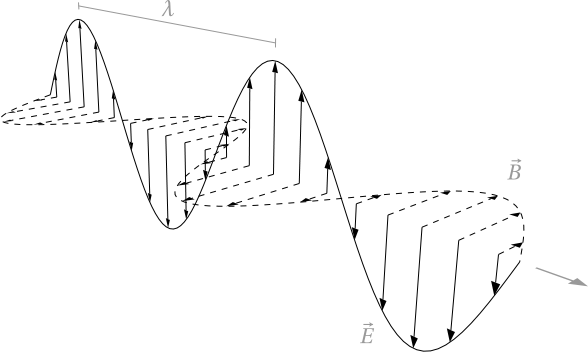Skip to main content

# 7.6: Electron Spin Resonance

Though less used than Nuclear Magnetic Resonance (NMR), Electron Paramagnetic Resonance (EPR) is a remarkably useful form of spectroscopy used to study molecules or atoms with an unpaired electron. It is less widely used than NMR because stable molecules often do not have unpaired electrons. However, EPR can be used analytically to observe labeled species in situ either biologically or in a chemical reaction.

## Introduction

Electron Paramagnetic Resonance (EPR), also known as Electron Spin Resonance (ESR). The sample is held in a very strong magnetic field, while electromagnetic (EM) radiation is applied monochromatically (Figure 1).Figure 1(3)-monochromatic electromagnetic beam

This portion of EPR is analogous to simple spectroscopy, where absorbance by the sample of a single or range of wavelengths of EM radiation is monitored by the end user ie absorbance. The unpaired electrons can either occupy +1/2 or -1/2 ms value (Figure 2). From here either the magnetic field "B0" is varied or the incident light is varied. Today most researchers adjust the EM radiation in the microwave region, the theory is the find the exact point where the electrons can jump from the less energetic ms=-1/2 to ms=+1/2. More electrons occupy the lower ms value (see Boltzmann Distribution).Figure 2: Resonance of a free electron.

Overall, there is an absorption of energy. This absorbance value, when paired with the associated wavelength can be used in the equation to generate a graph of showing how absorption relates to frequency or magnetic field.

$\Delta E=h\nu=g_e \beta_B B_0$

where ge equals to 2.0023193 for a free electron; $$\beta_B$$ is the Bohr magneton and is equal to 9.2740 * 10 -24 J T -1; and B0 indicates the external magnetic field.

## Theory

Like NMR, EPR can be used to observe the geometry of a molecule through its magnetic moment and the difference in electron and nucleus mass. EPR has mainly been used for the detection and study of free radical species, either in testing or anylytical experimentation. "Spin labeling" species of chemicals can be a powerfull technique for both quantification and investigation of otherwise invisible factors.

The EPR spectrum of a free electron, there will be only one line (one peak) observed. But for the EPR spetrum of hydrogen, there will be two lines (2 peaks) observed due to the fact that there is interaction between the nucleus and the unpaired electron. This is also called hyperfine splitting. The distance between two lines (two peaks) are called hyperfine splitting constant (A).

By using (2NI+1), we can calculate the components or number of hyperfine lines of a multiplet of a EPR transtion, where N indicates number of spin, I indicates number of equivalent nuclei. For example, for nitroxide radicals, the nuclear spin of 14N is 1, N=1, I=1, we have 2 x 1 + 1 = 3, which means that for a spin 1 nucleus splits the EPR transition into a triplet.

To absorb microwave, there must be unpaired electrons in the system. no EPR signal will be observed if the system contains only paired electrons since there will be no resonant absorption of microwave energy. Molecules such as NO, NO2, O2 do have unpaired electrons in groud states. EPR can be also performed on proteins with paramagnetic ions such as Mn2+, Fe3+ and Cu2+. Additionally, molecules containing stable nitroxide radicals such as 2,2,6,6-tetramethyl-1-piperidinyloxyl (TEMPO, Figure 3) and di-tert-butyl nitroxide radical.Figure 3-The nitroxide radical TEMPO

Examples of EPR spectra:Figure 4 -Stimulated EPR spectrum of CH3 radicalFigure 5 - Stimulated EPR spectrum of methoxymethl ( H2C(OCH3) )radical

## References

1. G. Maksina, Yu. S. Arkhangel'skaya and B. A. Dainyak. Russian State Medical University, Moscow. Translated from Meditsinskaya Tekhnika, No. 5, pp. 32–34, September–October, 1995.http://www.springerlink.com/content/n73312406t6941l4/fulltext.pdf
2. E.F. Block. The Role in Coherent Resonance in Human Fair: Part One-Electromagnetic and Gravity. December 2010. http://journalinformationalmedicine.org/cr1.htm
3. Thomas Engel, Gary Drobny and Philip Reid. Physical Chemistry for the Life Science.s 2008 Pearson Education, Inc. Upper Saddle River, NJ 07458. pp. 514-516
4. Cortel, Adolf. "Demonstrations on Paramagnetism with an Electronic Balance." J. Chem. Educ. 1998 75 61.
5. Geselbracht, Margaret J.; Cappellari, Ann M.; Ellis, Arthur B.; Rzeznik, Maria A.; Johnson, Brian J. "Rare Earth Iron Garnets: Their Synthesis and Magnetic Properties." J. Chem. Educ. 1994, 71, 696.
6. Shimada, Hiroshi; Yasuoka, Takashi; Mitsuzawa, Shunmei. "Observation of paramagnetic property of oxygen by simple method: A simple experiment for college chemistry and physics courses (TD)." J. Chem. Educ. 1990, 67, 63.